### Home > PC > Chapter 8 > Lesson 8.2.4 > Problem8-127

8-127.
1. Use properties of logs to solve each of the following equations. Use exact values. Homework Help ✎

1. log(x + 3) = 2

2. ln(x + 3) = 2

3. ln 3 + ln(x) = 4

4. ln(x + 4) − ln(x) = 2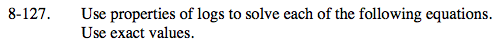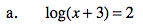10log(x+3) = 102
x + 3 = 100
x = 97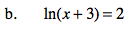elog(x+3) = e2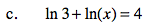ln(3x) = 4
eln(3x) = e4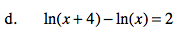$\text{ln}\frac{x+4}{x}=2$

$e^{\text{ln}\frac{x+4}{x}}=e^{2}$

$\frac{x+4}{x}=e^{2}$

x + 4 = xe2
xxe2 = −4
x(1 − e2) = −4

$x=\frac{-4}{1-e^{2}}\Rightarrow \frac{4}{e^{2}-1}$